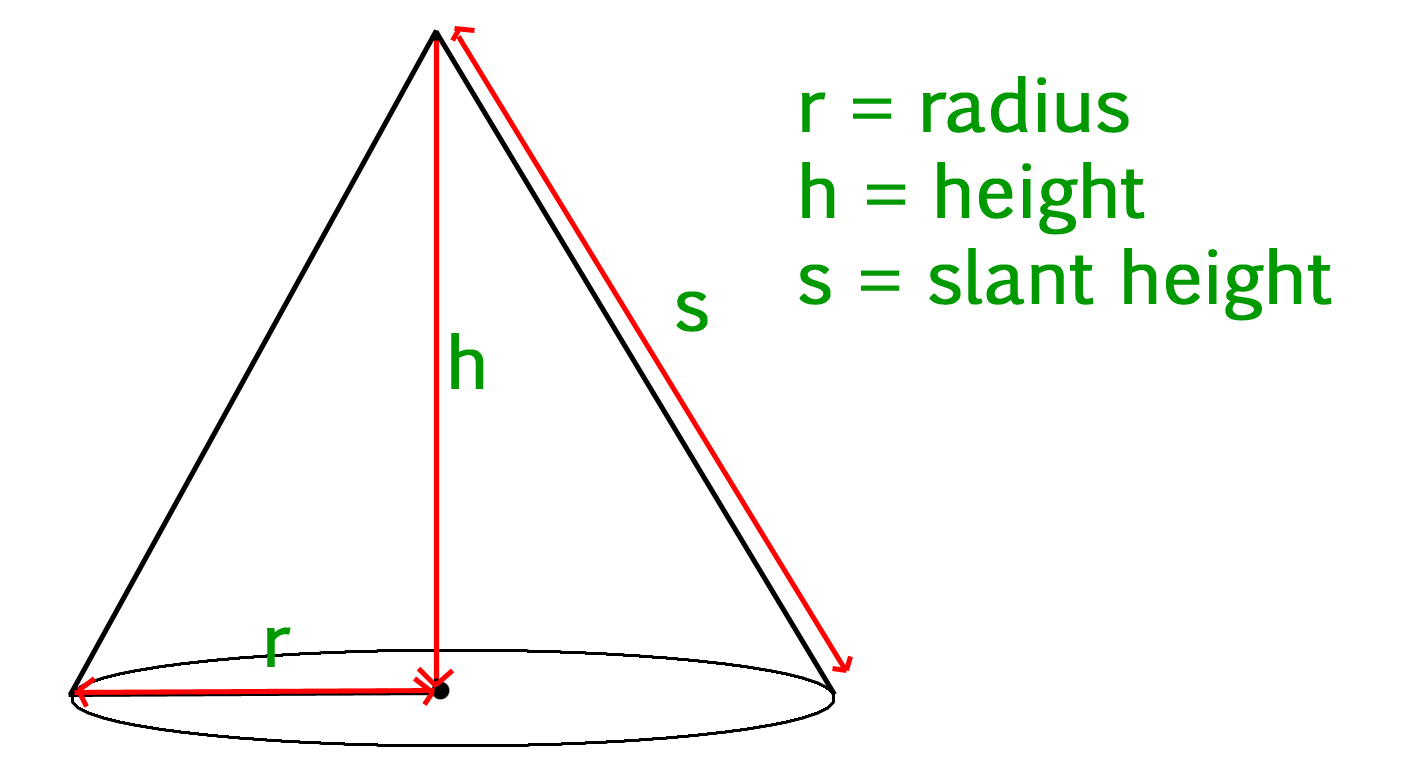Open in App
Not now

# Java Program to Find the Surface Area and Volume of a Cone

• Last Updated : 05 Sep, 2022

Given the dimensions of the cone, find the Surface area and Volume of a cone. The formula’s to calculate the area and volume are given below.

Cone

Cone is a three-dimensional geometric shape. It consists of a base having the shape of a circle and a curved side (the lateral surface) ending up in a tip called the apex or vertex.Surface Area of Cone = Area of cone + Area of Circle = pi * r * s + pi * r^2

Volume of Cone = 1/3(pi * r * r * h)

where r is the radius of the circular base, h is the height (the perpendicular distance from the base to the vertex) and s is the slant height of the cone.

Slant height (s) can be calculated using Pythagoras formula sqrt(r * r + h * h)

```Input :
slant_height = 13
height = 12
Output :
Volume Of Cone = 314.159
Surface Area Of Cone = 282.743

Input :
slant_height = 10
height = 8
Output :
Volume Of Cone = 301.593
Surface Area Of Cone = 301.593```

Approach :

• Given the dimensions of the cone, say radius R and height H of cone
• Find S = sqrt(R * R + H * H)
• Apply the above formulas

Example 1:

## Java

 `// Java Program to Find the Surface Area and Volume of a``// Cone` `import` `java.io.*;` `class` `GFG {``    ``public` `static` `void` `main(String[] args)``    ``{``        ``// specify radius and height of cone``        ``double` `R = ``6``, H = ``8``;` `        ``// calculate slant height S``        ``double` `S = Math.sqrt(R * R + H * H);` `        ``// calculate surface area of cone``        ``double` `SurfaceArea``            ``= (Math.PI * R * R) + (Math.PI * R * S);` `        ``// calculate volume of cone``        ``double` `Volume = (Math.PI * R * R * H) / ``3``;` `        ``System.out.println(``"Surface area of cone is : "``                           ``+ SurfaceArea);` `        ``System.out.println(``"Volume of cone is : "` `+ Volume);``    ``}``}`

Output

```Surface area of cone is : 301.59289474462014
Volume of cone is : 301.59289474462014```

Time Complexity : O(sqrt(R^2+H^2)) where r and h are the given radius and height of the cone, and sqrt function is being used

Auxiliary Space: O(1) because constant variables have been used

Example 2:

## Java

 `// Java Program to Find the Surface Area and Volume of a``// Cone` `import` `java.io.*;` `class` `GFG {``    ``public` `static` `void` `main(String[] args)``    ``{``        ``// specify radius and height of cone``        ``double` `R = ``3.42``, H = ``12``;` `        ``// calculate slant height S``        ``double` `S = Math.sqrt(R * R + H * H);` `        ``// calculate surface area of cone``        ``double` `SurfaceArea``            ``= (Math.PI * R * R) + (Math.PI * R * S);` `        ``// calculate volume of cone``        ``double` `Volume = (Math.PI * R * R * H) / ``3``;` `        ``System.out.println(``"Surface area of cone is : "``                           ``+ SurfaceArea);` `        ``System.out.println(``"Volume of cone is : "` `+ Volume);``    ``}``}`

Output

```Surface area of cone is : 170.81027853689216
Volume of cone is : 146.98129725379061```

Time Complexity : O(log(r2+h2)) where r and h are the given radius and height of the cone.
Auxiliary Space : O(1)

My Personal Notes arrow_drop_up